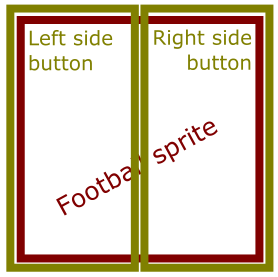# Set Velocity.X based on which part of sprite is clicked

## Recommended Posts

I am making a soccer keep up game, which consists solely of a ball that you click and have to prevent hitting the floor. So far I have got everything working, apart from the left/right movement of the ball. Currently, I have set it so when the ball is clicked, it is given a Y velocity making it go up in the air again. The issue is it goes straight up. I tried generating a random X velocity to make it also go left/right on click, but the problem is it is random. So if the ball is falling from left to right, clicking on the right side of the ball should make it go back left (as a ball would react in real life) but as it is currently random sometimes it will right instead.

I feel like I need to get the position of the actual click on the sprite, and from that determine which direction the ball should go. Unless there is a better way? I have included my code below:

``````var game = new Phaser.Game(800, 600, Phaser.AUTO, 'game-wrapper', { preload: preload, create: create, render:render });

var score = 0;
var text;

}

function create() {

text = game.add.text(100, 50, "Score: 0", { font: "32px Arial", fill: "#ff0000" });
text.anchor.set(0.5);

//  Enable p2 physics engine
game.physics.startSystem(Phaser.Physics.P2JS);

//Enable Impact Events for Collisions/Clicks etc
game.physics.p2.setImpactEvents(true);

//Set Background Color
game.stage.backgroundColor = '#009900';

//Set Physics - Gravity and Ball 'Weight'
game.physics.p2.gravity.y = 500;
game.physics.p2.restitution = 0.9;

//Add Ball, clip to circle, enable physics on ball
game.physics.p2.enable(ball);
ball.body.setCircle(80);

//Stop ball from colliding with world boundaries
ball.body.collideWorldBounds = false;

//Allow input on ball, and check for click input
ball.inputEnabled = true;

//Check location of ball to see if it is within boundaries, run function 'offScreen' if not
ball.checkWorldBounds = true;

}

function render() {

game.debug.spriteInputInfo(ball, 50, 500);

}

function clickDown(ball, pointer) {

var randX = Math.floor(Math.random()*300) + 1;
randX *= Math.floor(Math.random()*2) == 1 ? 1 : -1;

ball.body.velocity.y = -500;
ball.body.velocity.x = randX;

score += 1;
text.text = "Score: " + score;

}

function offScreen() {

}
``````

Any help would be appreciated!

Thanks##### Share on other sites

The simplest way of just having left/right detection I can think of is to have two buttons, each one is half as wide as the ball sprite, and attach them to the ball. Each button when pressed, gives a left/right velocity.Perhaps a more elegant solution would be when the ball is pressed, calculate if the input down X is more or less than the X of the center of the ball sprite. If input down X is greater than the center X of the ball sprite, the right half of the sprite was pressed. Else, must have been left side.

##### Share on other sites

Thanks for taking the time to answer in detail. Both good suggestions (in theory at least!). I will play around and see what I can get working!##### Share on other sites

The Phaser example Breakout game has a working example of the latter solution Arcanorum suggested, applying the difference between the clicked point and the center of the sprite to the velocity.

``````function ballHitPaddle (_ball, _paddle) {

var diff = 0;

{
//  Ball is on the left-hand side of the paddle
_ball.body.velocity.x = (-10 * diff);
}
{
//  Ball is on the right-hand side of the paddle
_ball.body.velocity.x = (10 * diff);
}
else
{
//  Ball is perfectly in the middle
//  Add a little random X to stop it bouncing straight up!
_ball.body.velocity.x = 2 + Math.random() * 8;
}

}``````